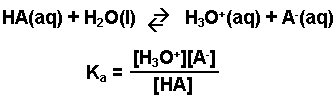## Determining cathode and anode from two half-reactions!

Regina Chi 2K
Posts: 51
Joined: Fri Sep 26, 2014 2:02 pm

### Determining cathode and anode from two half-reactions!

This is problem 8 from the 2014 practice midterm exam. But it can also be a very general question (does not have to apply to this specific problem). From two given half-reactions, how do we determine which is the anode and which is the cathode. I usually try to assign anodes and cathodes to get the most positive Ecell value; however, that does not seem to be the case every time because sometimes, the Ecell value will be negative (like in this problem). Also, I don't quite understand why we have to take the square root of K in order to get Ka. Thanks in advanced!

Sarah H Brown 1L
Posts: 56
Joined: Fri Sep 26, 2014 2:02 pm

### Re: Determining cathode and anode from two half-reactions!

There are a few rules when it comes to determining the anode and the cathode. When you are given a reaction or the question indicates reactions and products, you have to fit your redox equation to what is given in the question. For example, in question 8, it is asking for the Ka of HF which means HF must be the reactant. Therefore, you can write the dissociation equation:
HF <--> H+(aq) + F-(aq)
You know your half reactions must balance so that HF is a reactant and H+ and F- are products, so you have to fit the anode and cathode to these requirements, even if E0Cell is negative.
You take the square root of K because K (NOT Ka) = ([H+]2[F-]2)/[HF]2
Everything is squared! But you know that from the first balanced HF dissociation reaction you wrote, all of the coefficients are 1. Therefore, you can take the whole square root of the K expression to get the Ka for the non-redox equation HF <--> H+(aq) + F-(aq) (changing all of the exponents from 2 to 1). Ka = ([H+][F-])/[HF]

In a question where you are told it is a galvanic cell and you are given half reactions or couplets, then you make the most negative or smallest E0 value the anode so that E0cell is positive.

Regina Chi 2K
Posts: 51
Joined: Fri Sep 26, 2014 2:02 pm

### Re: Determining cathode and anode from two half-reactions!

Okay thanks, but why is it that for finding Ka, we have to make HF our reactant? I still don't think I really get that.

Sarah H Brown 1L
Posts: 56
Joined: Fri Sep 26, 2014 2:02 pm

### Re: Determining cathode and anode from two half-reactions!

So in 14A, we learned the general equation for a weak acid is

HA <--> H+ + A-

and so you know the general form for Ka is ([H+][A-])/[HA]

It can also be written as:if you want to explicitly include water and the hydronium ion.

It is a convention for weak acids. The strength of an acid is based on the dissociation of the H+ ion, and Ka is a measure of the strength of the acid. So you have to have H+ as a product because you are examining its formation.